Better diffs in pytest

How often do you compare dictionaries in (unit) tests? How do you handle diff outputs to make them as readable as possible?

Let's create a simple test.

def test_diff():
assert {"x": 1} == {"x": 2}

The default test summary looks like this: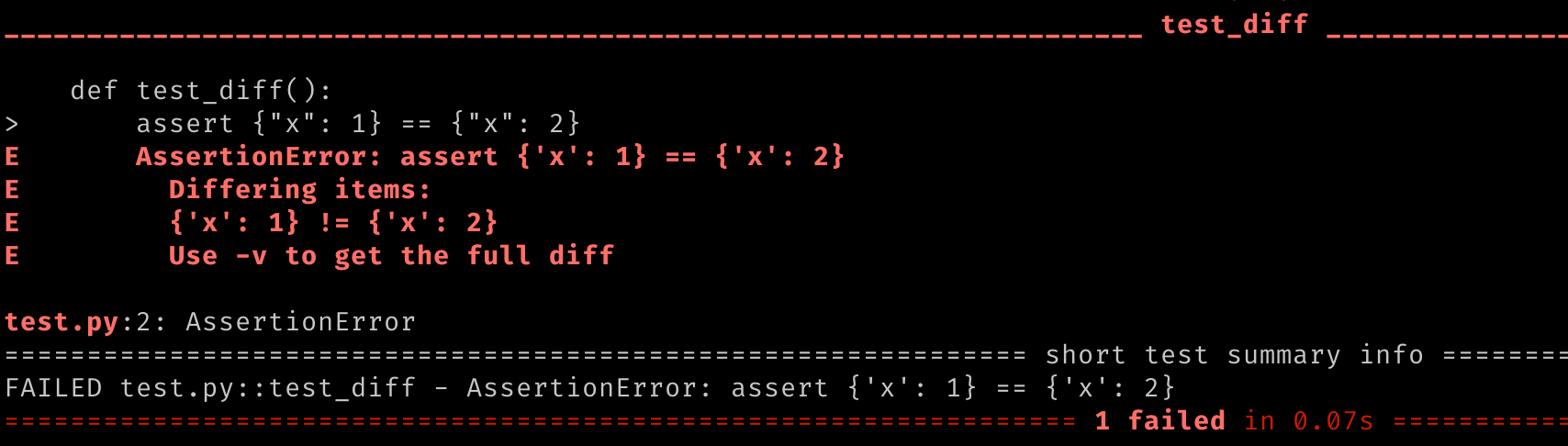The dictionaries are very small so we can see the difference without any issues. What if the dictionaries are bigger? Look at this example.

from faker import Faker

fake = Faker()
dict_1 = fake.pydict(nb_elements=10)
dict_2 = dict_1.copy()
dict_2[list(dict_1.keys())] = "Test 1"
dict_2[list(dict_1.keys())] = "Test 2"
dict_2[list(dict_1.keys())] = "Test 3"
dict_2[list(dict_1.keys())] = "Test 4"
dict_2[list(dict_1.keys())] = "Test 5"

def test_diff():
assert dict_1 == dict_2

The result.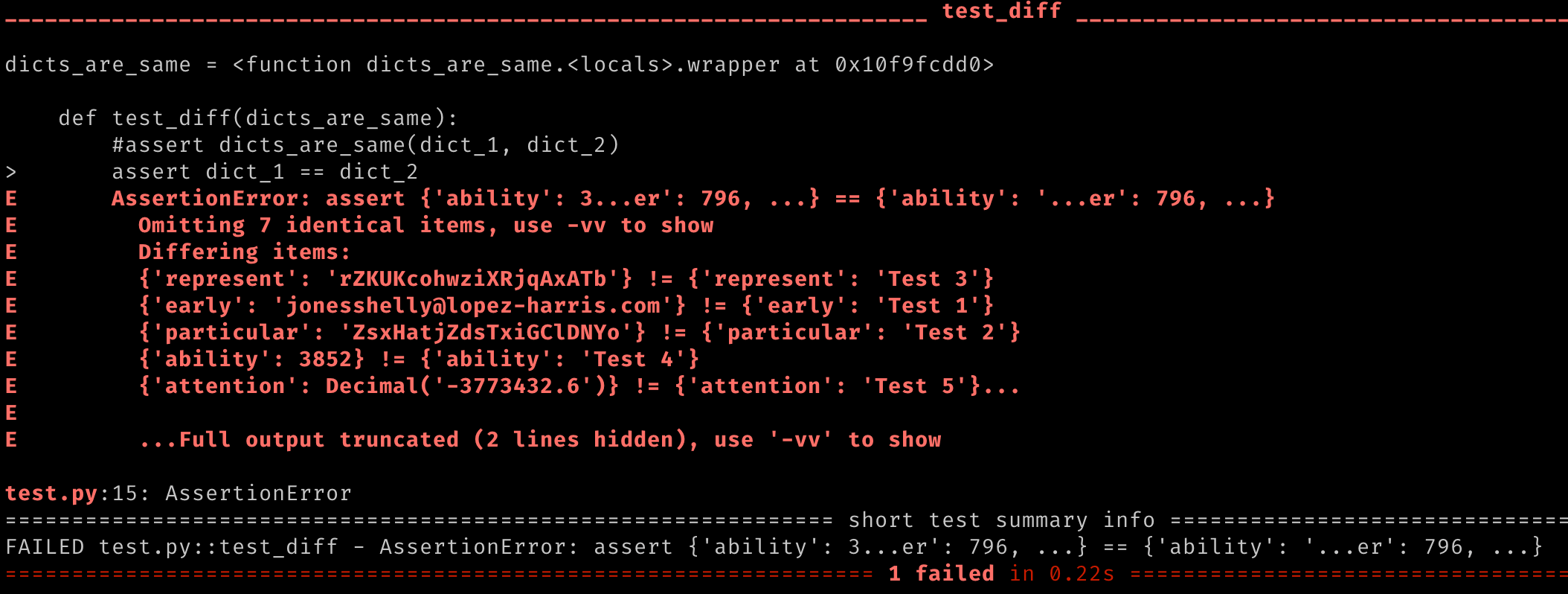It does not look good, right? We can see more or less what's wrong but it's not the easiest diff to read. We can try to use -vv option.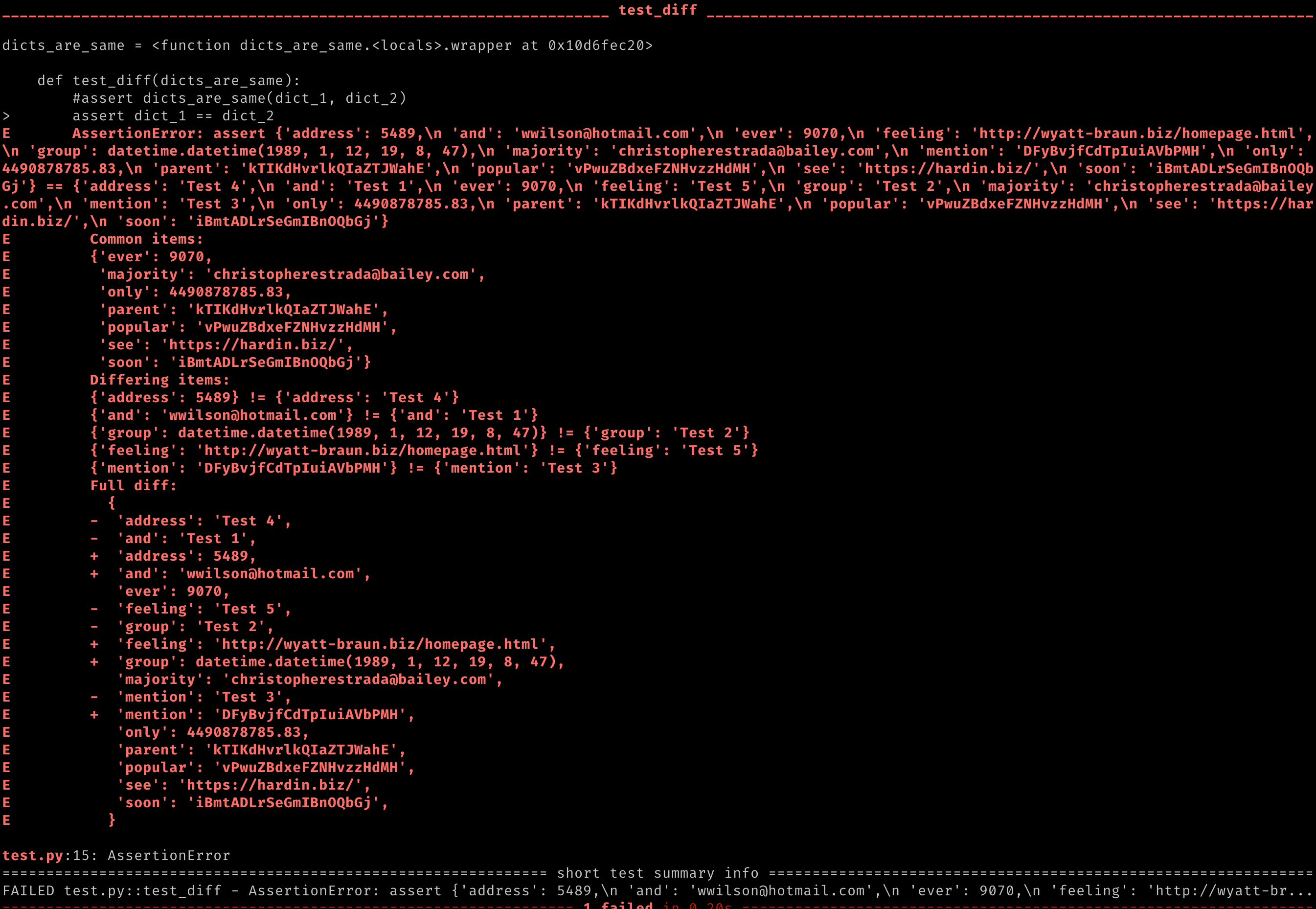How about now? We can see more details but still, it's not good enough!

Can we improve the output? Of course we can!

# Compare smaller chunks

It's doable, right? If you know what to expect, you can sort the dictionaries, iterate and compare step by step but... I don't think it's what we want to do in each test. I don't even want to write an example. Unit tests should be simple.

# dictdiffer (pytest-dictsdiff)

You can use dictdiffer and implement custom method or fixture to compare the dictionaries. You can also install pytest plugin, pytest-dictsdiff. It uses dictdiffer under the hood and provides ready to use fixture.

from faker import Faker

fake = Faker()
dict_1 = fake.pydict(nb_elements=10)
dict_2 = dict_1.copy()
dict_2[list(dict_1.keys())] = "Test 1"
dict_2[list(dict_1.keys())] = "Test 2"
dict_2[list(dict_1.keys())] = "Test 3"
dict_2[list(dict_1.keys())] = "Test 4"
dict_2[list(dict_1.keys())] = "Test 5"

def test_diff(dicts_are_same):
assert dicts_are_same(dict_1, dict_2)

The result.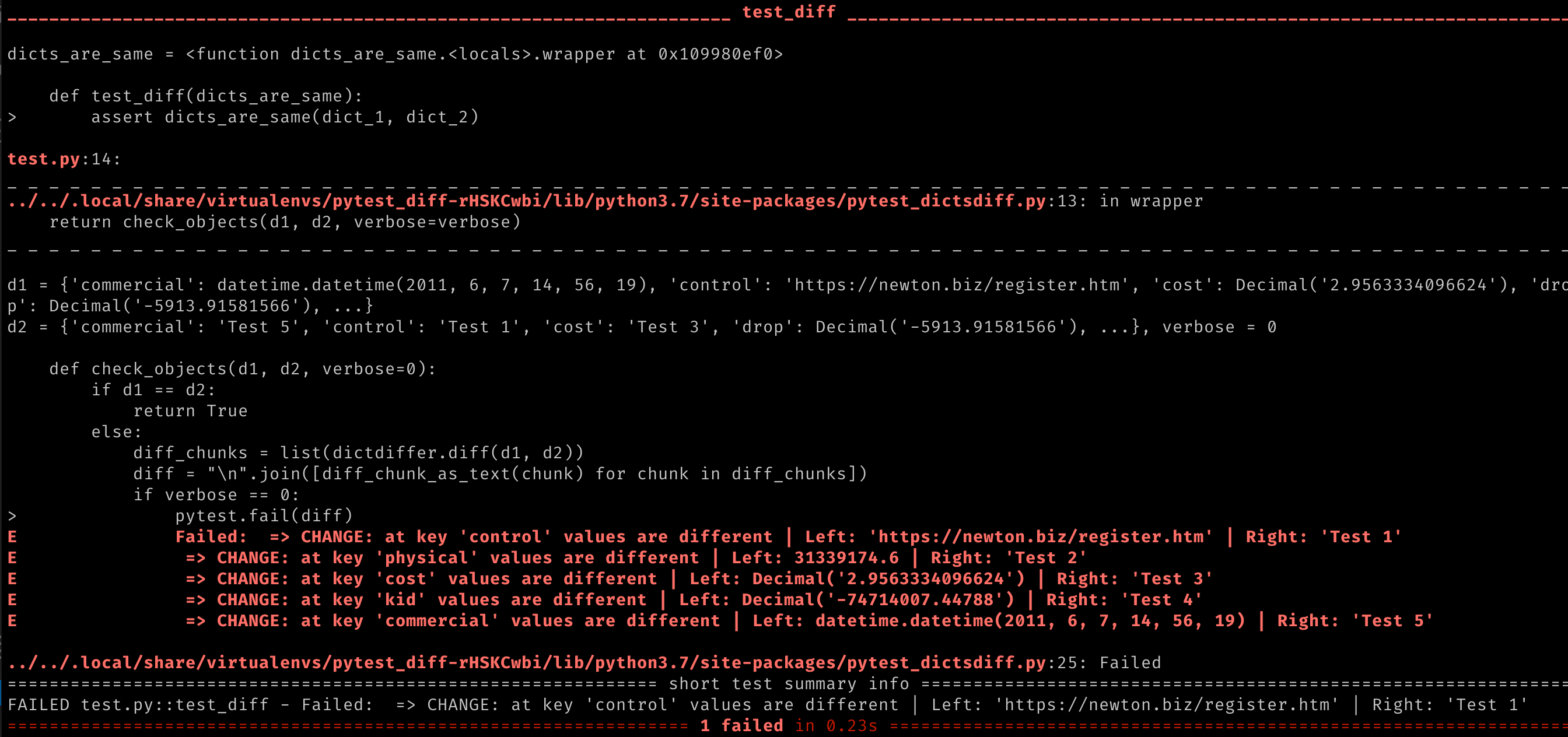It gives you information about differences between both dictionaries, line by line. It looks better than the default diffs but I would like to present a better option.

# pytest-icdiff

My favorite solution, pytest-icdiff. It displays a better diff summary and we don't need additional code!

Look at this. Use the same example as at the beginning.

from faker import Faker

fake = Faker()
dict_1 = fake.pydict(nb_elements=10)
dict_2 = dict_1.copy()
dict_2[list(dict_1.keys())] = "Test 1"
dict_2[list(dict_1.keys())] = "Test 2"
dict_2[list(dict_1.keys())] = "Test 3"
dict_2[list(dict_1.keys())] = "Test 4"
dict_2[list(dict_1.keys())] = "Test 5"

def test_diff():
assert dict_1 == dict_2

The result.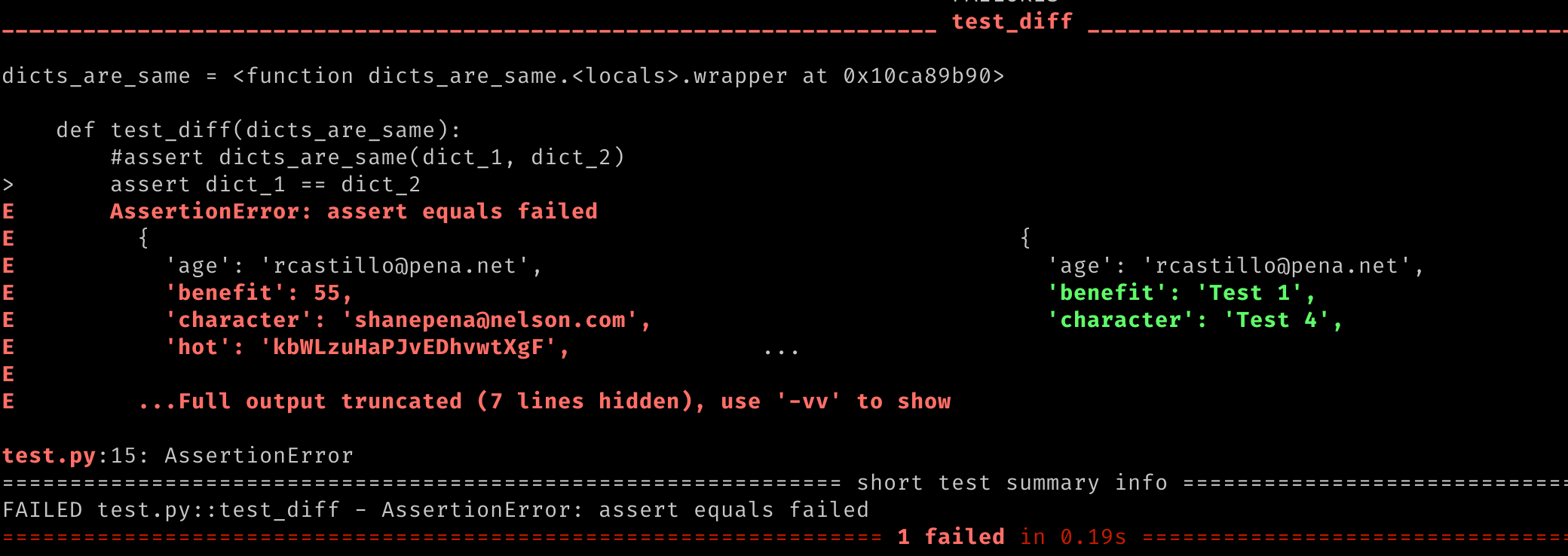Let's try to use -vv option.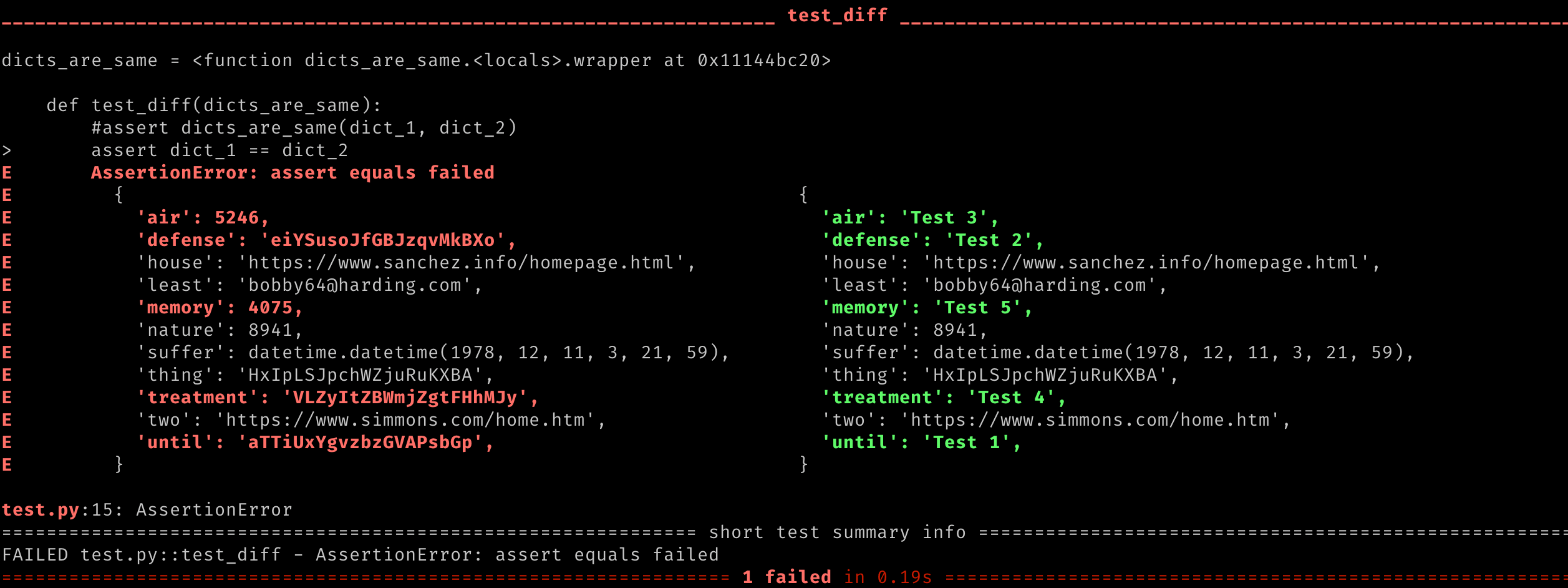How about you? Do you know better options?